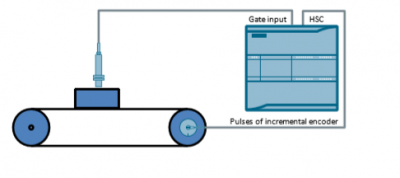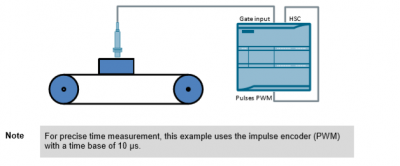In automation technology, there are many fast events that cannot be detected in the program cycle of the main OB. The high-speed counters (HSC) of the S7-1200 help you to process even those events.

Overview
This application example presents three possible applications for high-speed counters (HSC) of an S7-1200.

Measuring the speed in case of only one pulse or a few pulses per rotation
In the first example, the instruction “CTRL_HSC_EXT” is used to determine the speed of a rotary motion in case of only one pulse or a few pulses per rotation. Figure 1-1: Speed measurement in case of one pulse per rotationDetermining the length by means of a HW gate
The second example explains the use of a HW gate. In this case, the pulses of an incremental encoder are counted as long as a light barrier detects an object. The number of pulses is used to calculate the size of an object given a known shifting length per pulse. Figure 1-2: Determining the length by means of a HW gateDetermining the velocity by means of a HW gate
In the third example, the pulses of an incremental encoder (PWM) of the S7-1200 are counted by means of the HW gate as long as a light barrier detects an object. The number of pulses and the cycle time of the PWM signal are used to determine the duration of the HIGH signal at the HW gate. The velocity will be calculated from the duration and the defined known size of an object. Figure 1-3: Determining the velocity by means of a HW gate
OthersComponents used
This application example has been created with the following hardware and software components:Engineering: Speed measurement
The example for measuring the speed in case of only one pulse or a few pulses per rotation has been realized in the STEP 7 project “Ex01_Speed”.

Hardware setup
Figure 2-1: Hardware setup for speed measurement